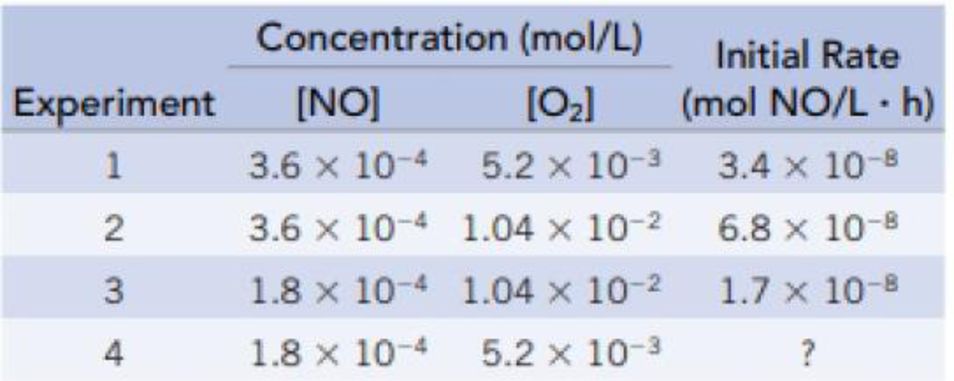# Data for the reaction NO(g) + ½ O 2 (g) → NO 2 (g) are given (for a particular temperature) in the table. (a) What is the rate law for this reaction? (b) What is the rate constant for the reaction? (c) What is the initial rate of the reaction in experiment 4?### Chemistry & Chemical Reactivity

9th Edition
John C. Kotz + 3 others
Publisher: Cengage Learning
ISBN: 9781133949640

#### Solutions

Chapter
Section### Chemistry & Chemical Reactivity

9th Edition
John C. Kotz + 3 others
Publisher: Cengage Learning
ISBN: 9781133949640
Chapter 14, Problem 13PS
Textbook Problem
58 views

## Data for the reaction NO(g) + ½ O2(g) → NO2(g) are given (for a particular temperature) in the table.(a) What is the rate law for this reaction? (b) What is the rate constant for the reaction? (c) What is the initial rate of the reaction in experiment 4?

(a)

Interpretation Introduction

Interpretation: The rate law for this reaction has to be given.

Concept introduction:

Rate law or rate equation: The relationship between the reactant concentrations and reaction rate is expressed by an equation.

aA + bBxXRate of reaction = k [A]m[B]n

Order of a reaction: The order of a reaction with respect to a particular reactant is the exponent of its concentration term in the rate law expression, and the overall reaction order is the sum of the exponents on all concentration terms.

Rate constant, k: It is a proportionality constant that relates rate and concentration at a given temperature.

### Explanation of Solution

The rate law for this reaction is calculated as,

Reaction Rate = k [NO]m[O2]n,where m, and n are orders of the reactants.Givenreaction:  NO(g) + 1/2 O2(g)®NO2(g)Findorderofthereaction:_Comparingfirsttwoexperiments2and3,rate2=[NO]m[O2]n, rate 2 = 6.8×10-8 mol/L.hrate3 = k [NO]m[O2]n, rate 3 = 1.7×10-8 mol/L.hrate 2rate3 = k [NO]m[O2]nk [NO]m[O2]n6.8×10-8 mol/L.h1.7×10-8 mol/L.h=(3.6×10-4)m(1.04×10-2)n(1.8×10-4)m(1.04×10-2)n 4 = (2)mm = 2Comparinglasttwoexperiments1 and2,rate1 =[NO]m[O2]n,rate 1 = 3

(b)

Interpretation Introduction

Interpretation:

The rate constant for the reaction has to be given.

Concept introduction:

Rate law or rate equation: The relationship between the reactant concentrations and reaction rate is expressed by an equation.

aA + bBxXRate of reaction = k [A]m[B]n

Order of a reaction: The order of a reaction with respect to a particular reactant is the exponent of its concentration term in the rate law expression, and the overall reaction order is the sum of the exponents on all concentration terms.

Rate constant, k: It is a proportionality constant that relates rate and concentration at a given temperature.

(c)

Interpretation Introduction

Interpretation: The rate law for this reaction has to be given.

Concept introduction:

Rate law or rate equation: The relationship between the reactant concentrations and reaction rate is expressed by an equation.

aA + bBxXRate of reaction = k [A]m[B]n

Order of a reaction: The order of a reaction with respect to a particular reactant is the exponent of its concentration term in the rate law expression, and the overall reaction order is the sum of the exponents on all concentration terms.

Rate constant, k: It is a proportionality constant that relates rate and concentration at a given temperature.

### Still sussing out bartleby?

Check out a sample textbook solution.

See a sample solution

#### The Solution to Your Study Problems

Bartleby provides explanations to thousands of textbook problems written by our experts, many with advanced degrees!

Get Started

Find more solutions based on key concepts
Water from public water systems requires frequent borne testing for microorganisms. is less healthful than bott...

Nutrition: Concepts and Controversies - Standalone book (MindTap Course List)

A series RLC circuit has a resistance of 22.0 and an impedance of 80.0 . If the rms voltage applied to the cir...

Physics for Scientists and Engineers, Technology Update (No access codes included)

Whats a limiting factor? Can you provide an example?

Oceanography: An Invitation To Marine Science, Loose-leaf Versin